# MCQs on Electrical And Electronics Measurements

##### Page 10 of 19. Go to page 1 2 3 4 5 6 7 8 9 10 11 12 13 14 15 16 17 18 19
01․ The meter constant of a single phase 240 V induction watthour meter is 400 revolutions per KWh. The speed of the meter disc for a current of 10 Amperes of 0.8 p.f lagging will be
21.1 rpm.
18.2 rpm.
16.02 rpm.
12.8 rpm.

n = K × power × time in minutes = {400 × 240 × 10 × 0.8/1000} × (1/60) rpm = 12.8 rpm.

02․ In DC potentiometer measurments, a second reading is often taken after reversing the polarities of the DC supply and the unknown voltage and the average of the two reading is taken. This is with a view of eliminate the effects of
Stray magnetic fields.
Ripples of the DC supply.
Errorneous standardisation.
Stray thermal emfs.

Error in the potentiometer is due to thermoelectric EMFs set up at junctions of dissimilar metals and also by the heat from the operator's hand during adjustment of the working parts of the potentiometer. Reversing of polarity and taking reading will give the error in the negative direction. Suppose the reading with error due to one direction is +ΔR and in the other direction is -ΔR, then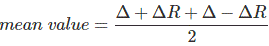Which is actual value.

03․ In the bridge given in the figure, the reading of the high impedance voltmeter is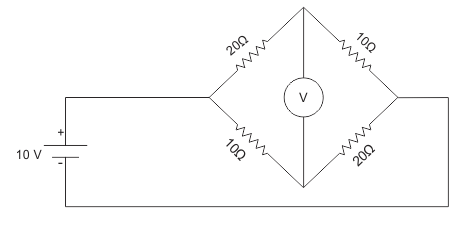Zero.
6.66 V.
4.20 V.
- 3.33 V.

The bridge is not balanced. So the equivalent resistance of the circuit is (10 + 20) parallel (10 + 20) = 15 Ohm. Current in each branch is I = 10/30 = 1/3 A. Voltage reading of voltmeter is – 20 × 1/3+ 10 × 1/3= - 3.33 V.

04․ A CRO probe has an impedance of 500 kΩ in parallel with a capacitance of 10 pF. The probe is used to measure the voltage between P and Q as shown in figure. The measured voltage will be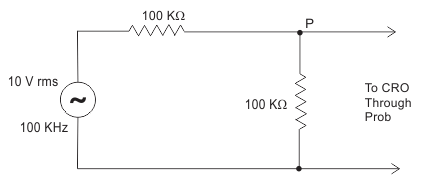3.53 V.
4.38 V.
4.54 V.
5 V.

In the following configuration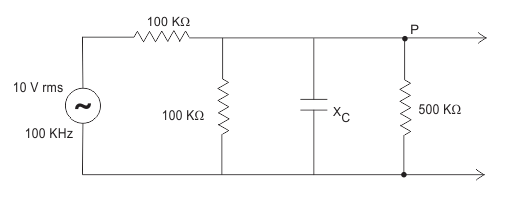Reactance (XC) = 1/jωC = 159 kΩ. Writing node equation at P, (VP – 10)/100 + VP /100 + VP /(500 - j/159) = 0. VP = {10/2.28} = 4.38 V.

05․ Consider the following statement - 1. The compensating coil of a low power factor wattmeter compensates the effect of the impedance of the current coil. 2. The compensating coil of a low power factor wattmeter compensates the effect of the impedance of the voltage coil circuit.
1 is true but 2 is false.
1 is false but 2 is true.
Both 1 and 2 are true.
Both 1 and 2 are false.

Compensating coil of a low power factor wattmeter compensating the effect of the impedance of pressure coil or voltage coil circuit.

06․ The measurment of very low and very high frequencies is invariably done using a frequency counter in which one of the following?
Period and frequency measurement modes respectively.
Frequency and period measurment modes, respectively.
Frequency measurment modes only.
Period measurment modes only.

Low frequency measurment in frequency mode gives low accuracy. So period measurement is used.

07․ What is the approximate input impedance of a CRO?
0
1 MΩ
10 MΩ
100 MΩ

CRO has input impedance in the range of 1MΩ.

08․ Piezoelectronic accelerometer
Has a low natural frequency.
Should be used for low frequency.
Should be used for high frequencies above 100 Hz.
Should use a monitoring source at low input impedance.

A piezoelectric accelerometer is an accelerometer that employs the piezoelectric effect of certain materials to measure dynamic changes in mechanical variable (e.g. acceleration, vibration and mechanical shock). It is generally used for low frequencies.

09․ A slide wire potentiometer has 10 wires of 1 m each. With the help of a standard voltage source of 1.018 V it is standardised by keeping the jockey at 101.8 cm. If the resistance of the potentiometer wires is 1000 ohm, then the value of the working current will be
0.5 mA.
1mA.
10 mA.
100 mA.

Total length of the slide wire = 1000 cm. Total resistance of the slide wire = 1000 ohm. Resistance of 101.8 cm segment of the wire = 101.8 ohm. This corresponds to a voltage of 1.018 V. Therefore current = 1.018/101.8= 10 mA.

10․ A galvanometer with a full scale current of 10 mA has a resistance of 1000 Ω. The multiplying power (the ratio of measured current to galvanometer current) of 100 Ω shunt with this galvanometer is
11.
110.
10.
100.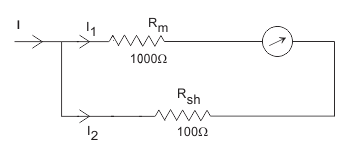We have to obtain n = I/I1. Here, {I1/I2} = RSh / Rm. I1 + I2 = I. N = 1+ I2/I1 = 1+10 = 11.

<<<89101112>>>